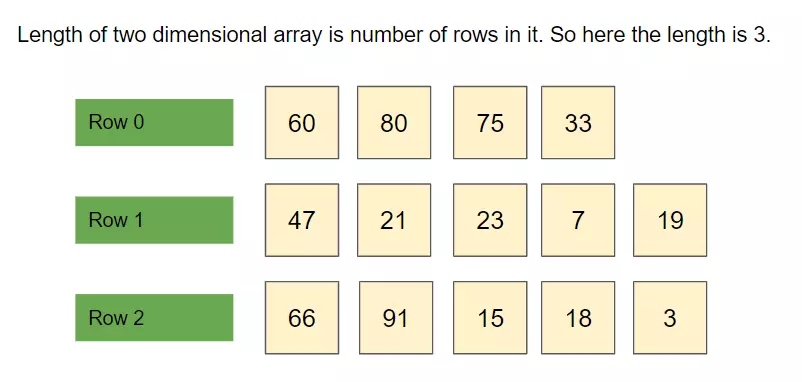# How to get the length of 2d array in Java

The length of 2d array in Java is the number of rows present in it. We check for the number of rows because they are fixed. Columns may vary per row, hence we cannot rely on that. Thus to determine the length of the two-dimensional array we count the rows in it.

Two-dimensional array is a set of rows and columns. Each row may have the same or different numbers of columns in it.

Things will become clear if you understand the below diagram.## Program to Get Two Dimensional Array length in Java

Let’s have a look at the program now.

```public class Demo {
public static void main(String[] args) {
// declare two dimensional array
int[][] elements = {
{ 60, 80, 75, 33 },
{ 47, 21, 23, 7, 19 },
{ 66, 91, 15, 18, 3 }
};

System.out.println("Length of 2d Array is : " + elements.length); // 3
System.out.println("*Size of*");
System.out.println("1st row : " + elements.length); // 4
System.out.println("2nd row : " + elements.length); // 5
System.out.println("3rd row : " + elements.length); // 5
}
}
```

Output:

```Length of 2d Array is : 3
*Size of*
1st row : 4
2nd row : 5
3rd row : 5
```

Explanation:

1. As a first step, we declared a two-dimensional array.
2. We used elements.length to find its size.
3. Next, we used elements.length to find the number of columns per row.
4. Array index always starts with 0 in Java.

Though finding the length is a trivial operation but it is a very useful one. From the output, you can figure out that the number of columns may differ per row.

I hope you understood the concept well. If you liked the article, please share it.

Categories: Java